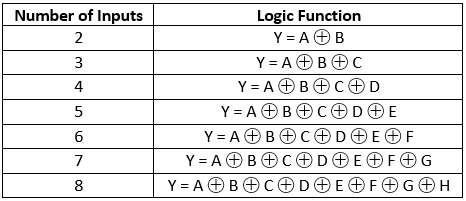# XOR

Digital XOR is an XOR logic gate with a number of inputs. The output Y is 1 if an odd number of the inputs are 1. Y is 0 if an even number of the inputs are 1.

Logic function